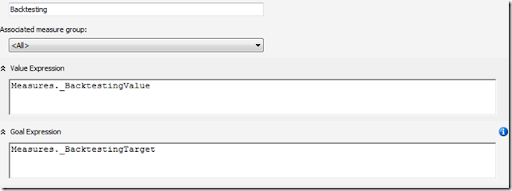## Monday, December 29, 2008

### EXISTING and query context

There had been some posts discussing the multi select problems in MDX. If, for example, you have a calculated measure using CURRENTMEMBER, the measure might not be computable when selecting a set as a WHERE condition. You can take a look at Mosha's blog for more information on this topic (see http://www.sqljunkies.com/WebLog/mosha/archive/2005/11/18/multiselect_friendly_mdx.aspx). Usually you can avoid the pitfalls of the multi select be changing the definition of your computed measures. The EXISTING operator is very helpful in this case. For this entry I concentrate on the EXISTING operator and the pitfalls you are facing.

To illustrate the problem, here's a simple example:

WITH
MEMBER MyCounter AS
count(
Filter((
[Date].[Calendar].[Date]),
([Measures].[Gross Profit Margin] > 0.42)))
MEMBER NumDays AS
count((
[Date].[Calendar].[Date]))
SELECT
{
NumDays,
MyCounter
} ON 0

The above MDX defines a measure (MyCounter) that counts the days for which the gross profit margin (in total) was above 42%. It also returns the number of the days selected. Running the query in the Adventure Works database returns something like this:

So there are 1158 days in the Date attribute und 249 of them are having a gross profit margin above 42%.

Please note that it's much better to rewrite the Count(Filter(...)) to a Sum(...) expression as shown below as this performs better on SQL Server 2008 Analysis Services. Mosha wrote an excellent entry on SQL Server 2008 block mode computations which can be found here: http://sqljunkies.com/WebLog/mosha/archive/2007/11/23/92198.aspx

Sum((
[Date].[Calendar].[Date]),
iif(
([Measures].[Gross Profit Margin] > 0.42),1,0))

For this post however I keep the Count(Filter(...)) as I think it's easier to read.

Up till now there hasn't been anything special. But now we're going to add a WHERE statement to the query above:

WHERE
[Date].[Calendar].[Calendar Year].&

If you are not familiar with MDX set operations you might expect a different query result after adding the WHERE condition. But this is not correct. The query give the same result, it seems to completely ignore our condition. The reason for that is that set operations like above are not aware of the current context defined by our WHERE condition.

This is the reason why in SQL Server 2005 the new EXISTING operator was added. The EXISTING operator forces the set operation to be computed inside the current context. So we're simply adding this operator to our query like this:

WITH
MEMBER MyCounter AS
count(
Filter((EXISTING
[Date].[Calendar].[Date]),
([Measures].[Gross Profit Margin] > 0.42)))
MEMBER NumDays AS
count((EXISTING
[Date].[Calendar].[Date]))
SELECT
{
NumDays,
MyCounter
} ON 0
WHERE
[Date].[Calendar].[Calendar Year].&

With this easy change to our query we get the desired result:

For example think of a KPI or calculated measure. Without the EXISTING operator the calculation could be wrong when selecting multiple days but with this easy change everything seems to be fine. But it's not that easy. To illustrate the problem let's turn our computation into a cube script calculated member and access this calculation by Excel 2007:

CREATE MEMBER CURRENTCUBE.[MEASURES].MyCounter AS
count(
Filter((EXISTING
[Date].[Calendar].[Date]),
([Measures].[Gross Profit Margin] > 0.42)));

CREATE MEMBER CURRENTCUBE.[MEASURES].NumDays AS
count((EXISTING
[Date].[Calendar].[Date]));

Now when accessing this member in Excel 2007 you'll see that the EXISTING fails if more than one day is selected as shown below:

While the calculation works fine as long there is only one day selected, it seems to be ignored when there is more than one element selected:

(The value 249 is the value from our first computation that had no WHERE statement at all)

The reason for this behavior is that Excel 2007 uses subcube queries in order to improve query performance. And for some reason, EXISTING doesn't work in the subcube scope. The query that was executed by Excel can easily be grabbed using the SQL Profiler). By the way, another cool way of capturing this query in Excel 2007 is to write a macro, as shown by Marco Russo in his blog.

SELECT
FROM
(
SELECT
{
[Date].[Calendar].[Date].&,
[Date].[Calendar].[Date].&
} ON COLUMNS
)
WHERE
[Measures].[MyCounter]

The fact that EXISTING doesn't really work with subcubes is somehow fatal as we like to design our cube calculations in a way they are compatible with all kinds of clients. The problem is that errors may not be easy to see and could be misleading.

So finally, even with the EXISTING keyword, multiselects are still an issue to really take care of in MS OLAP.

## Friday, December 5, 2008

### Formatting actual and goal for a KPI

Being able to specify the preferred format for measures and calculated measures in SSAS is a great feature since you just do the definition once and clients can leverage this central definition.

But for a KPI expression (value, goal, status, trend) it's not directly possible to specify the format. But there is still a simple trick to do so: You just have to define the calculation itself as a calculated member within your cube script like this:

create
member currentcube.Measures._BacktestingTotal as 100, visible=0

member currentcube.Measures._BacktestingValue as 5, visible=0 , format_string = "0.00%"

member currentcube.Measures._BacktestingTarget as 5, visible=0 , format_string = "0.00%"

I removed the real computation formulas for the three values and replaced them by constants because they would simply be confusing. But you can see how the format string is applied here. These members shouldn't be visible, so I set visible=0.

In our KPI definition you can simply point the expression to the calculated member like this:By doing so, the format of the calculated member defines the format for the KPI value, target etc.